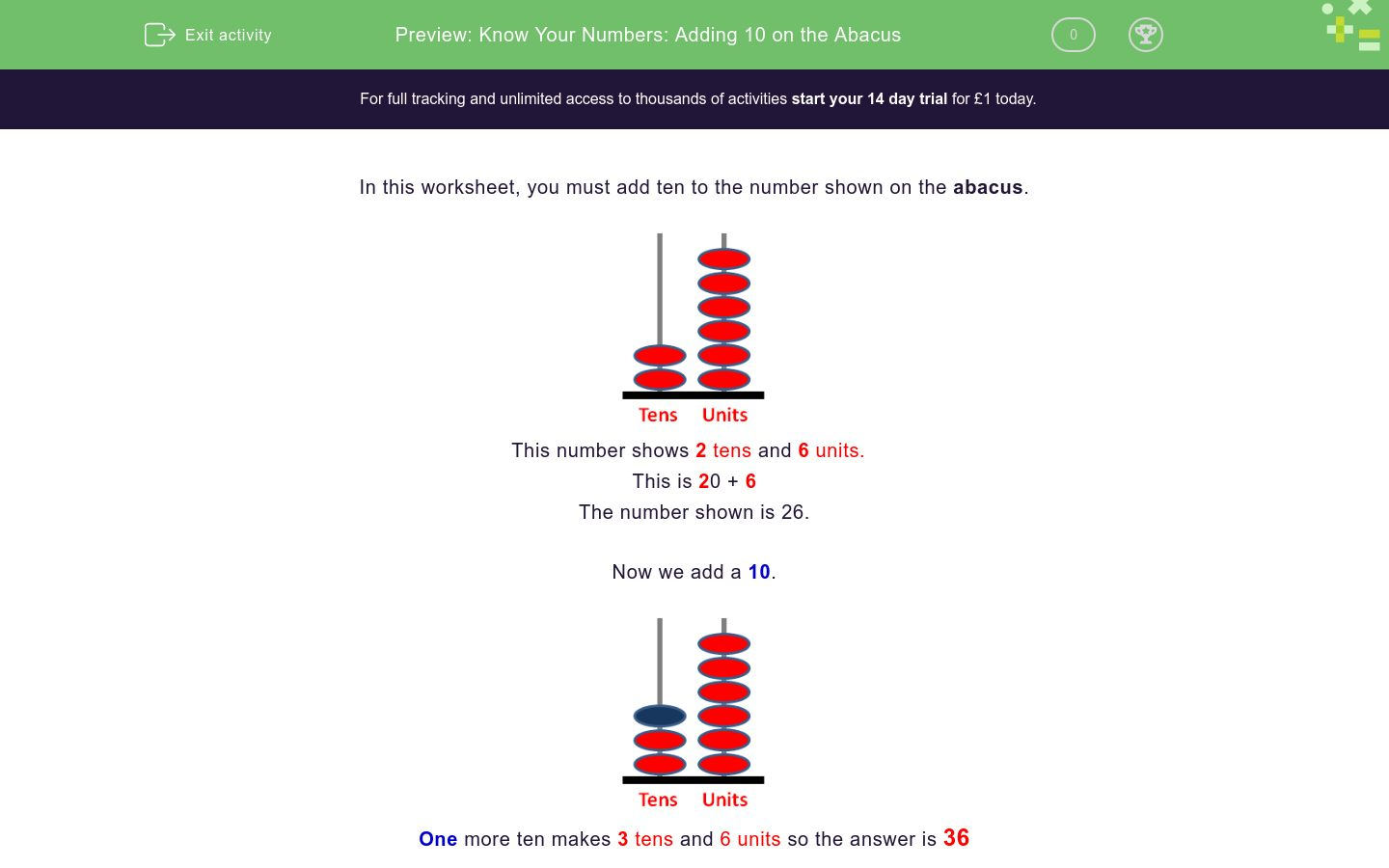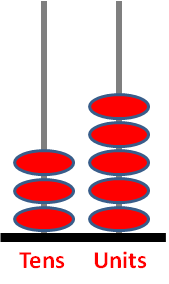### Affordable pricing from £10/month

In this worksheet, students add 10 to the number shown on the tens and ones abacus.Key stage:

Curriculum topic:

Curriculum subtopic:

Difficulty level:### QUESTION 1 of 10

In this worksheet, you must add ten to the number shown on the abacus.This number shows 2 tens and 6 units.

This is 20 + 6

The number shown is 26.One more ten makes 3 tens and 6 units so the answer is 36

Add 10 to the number shown on this abacus.Add 10 to the number shown on this abacus.Add 10 to the number shown on this abacus.Add 10 to the number shown on this abacus.Add 10 to the number shown on this abacus.Add 10 to the number shown on this abacus.Add 10 to the number shown on this abacus.Add 10 to the number shown on this abacus.Add 10 to the number shown on this abacus.Add 10 to the number shown on this abacus.• Question 1

Add 10 to the number shown on this abacus.53
EDDIE SAYS
4 tens + 1 ten = 5 tens
5 tens = 50
3 units = 3
50 + 3 = 53
• Question 2

Add 10 to the number shown on this abacus.31
EDDIE SAYS
2 tens + 1 ten = 3 tens
3 tens = 30
1 unit = 1
30 + 1 = 31
• Question 3

Add 10 to the number shown on this abacus.30
EDDIE SAYS
2 tens + 1 ten = 3 tens
3 tens = 30
(There are no units.)
• Question 4

Add 10 to the number shown on this abacus.14
EDDIE SAYS
0 tens + 1 ten = 1 ten
1 ten = 10
4 units = 4
10 + 4 = 14
• Question 5

Add 10 to the number shown on this abacus.45
EDDIE SAYS
3 tens + 1 ten = 4 tens
4 tens = 40
5 units = 5
40 + 5 = 45
• Question 6

Add 10 to the number shown on this abacus.36
EDDIE SAYS
2 tens + 1 ten = 3 tens
3 tens = 30
6 units = 6
30 + 6 = 36
• Question 7

Add 10 to the number shown on this abacus.93
EDDIE SAYS
8 tens + 1 ten = 9 tens
9 tens = 90
3 units = 3
90 + 3 = 93
• Question 8

Add 10 to the number shown on this abacus.48
EDDIE SAYS
3 tens + 1 ten = 4 tens
4 tens = 40
8 units = 8
40 + 8 = 48
• Question 9

Add 10 to the number shown on this abacus.33
EDDIE SAYS
2 tens + 1 ten = 3 tens
3 tens = 30
3 units = 3
30 + 3 = 33
• Question 10

Add 10 to the number shown on this abacus.85
EDDIE SAYS
7 tens + 1 ten = 8 tens
8 tens = 80
5 units = 5
80 + 5 = 85
---- OR ----

Sign up for a £1 trial so you can track and measure your child's progress on this activity.

### What is EdPlace?

We're your National Curriculum aligned online education content provider helping each child succeed in English, maths and science from year 1 to GCSE. With an EdPlace account you’ll be able to track and measure progress, helping each child achieve their best. We build confidence and attainment by personalising each child’s learning at a level that suits them.